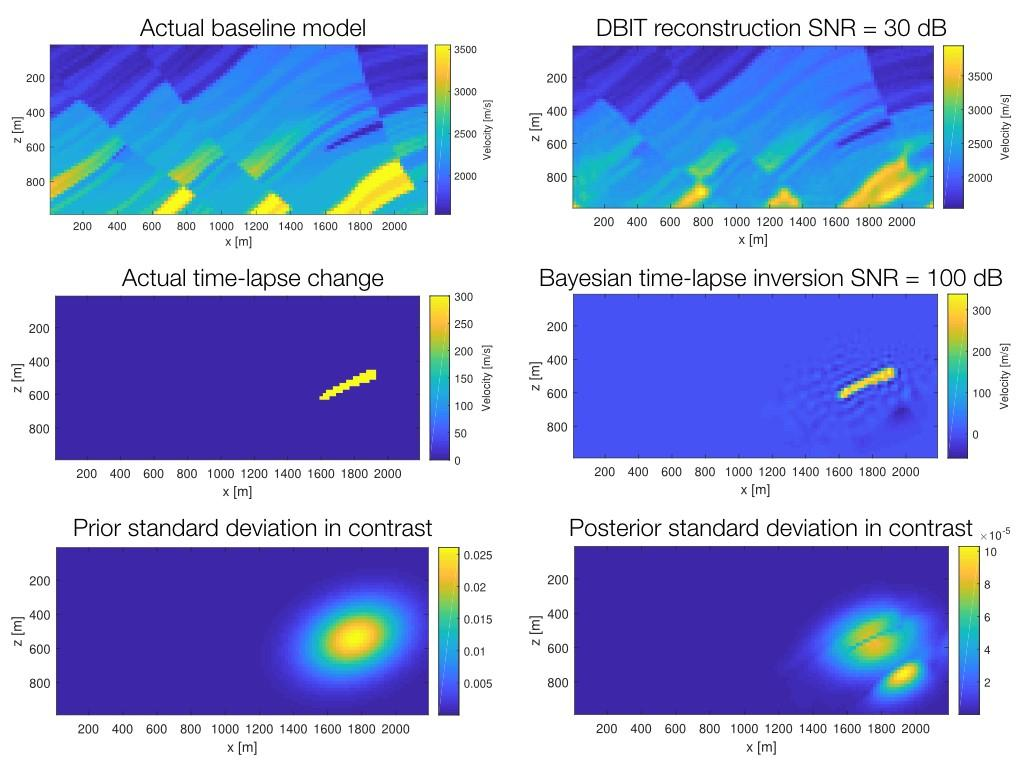# Lars André Fardal Refsland - Inversion of time-lapse seismic waveform data using integral equation methods

Lars Andreé Fardal Refsland - tok sin mastergrad 15. juni 2018 med tittelen: Inversion of time-lapse seismic waveform data using integral equation methodsFigure 1: Actual and inverted baseline and time-lapse models. Upper left figure shows the actual velocity model of the baseline model. Upper right figure shows the inverted results of the baseline model using the DBIT method. Middle left figure shows the actual time-lapse (change) introduced into the actual baseline model, middle right figure shows the corresponding inverted time-lapse change with data contaminated with noise and a corresponding signal-to-noise ratio of 100 dB. Lower left figure shows the prior standard deviation, containing prior information about location of the reservoir and size of expected change (in contrast). Lower right figure shows the uncertainties of the reconstructed time-lapse effect.
Foto/ill.:
Geo, UiB

## Hovedinnhold

Abstract
Time-lapse seismic data are considered a valuable tool for monitoring hydrocarbon reservoirs. Since the changes in elastic parameters associated with production of hydrocarbons, or injection of CO2 into a reservoir, tend to be relatively small compared to that of the geological surroundings, scattering integral equation methods have been chosen for the task of modelling time-lapse seismic data. Full waveform inversion (FWI) is a comprehensive imaging technique that employs all of the information contained in seismic data, including travel times, amplitudes, internal multiples and diffractions. This was first performed using the distorted Born iterative T-matrix (DBIT) method, an iterative inversion approach that solved linear inverse problems at every iteration. This method has been performed on two models. Two time-lapse experiments was performed using a sequential strategy. First a time-lapse effect was reconstructed on a simple 2D reservoir model. This was followed by a demonstration of the time-lapse inversion of a re-sampled version of the Marmousi model. For future applications in ensemble based history matching, a linear Bayesian approach, using the distorted Born approximation, has been examined. The inversion process was linearised around a reconstructed inhomogeneous baseline model, for which Green’s functions were calculated. The distorted Born approximation was then used with explicit Bayesian expressions, resulting in a maximum a posteriori (MAP) solution of time-lapse effects. Posterior uncertainties were also obtained. The Bayesian inversion method has been tested in two different time-lapse scenarios. First the Marmousi model was introduced with a relatively large time-lapse velocity change. The linear Bayesian time-lapse inversion was also tested on a smaller contrast. The DBIT method did prove to be able to reconstruct both models to a satisfying degree. The linear Bayesian time-lapse inversion was also able to reconstruct a time-lapse effect in the Marmousi model, even with a high level of noise.